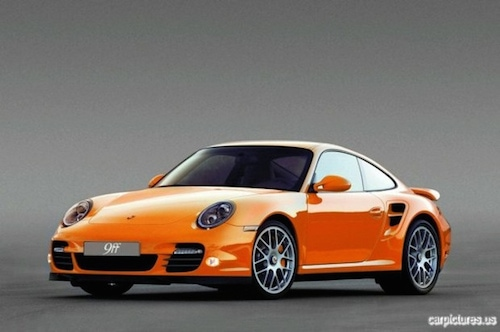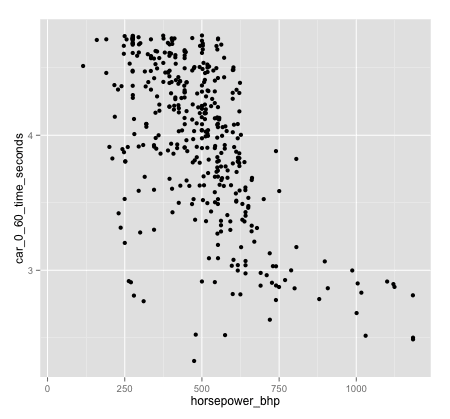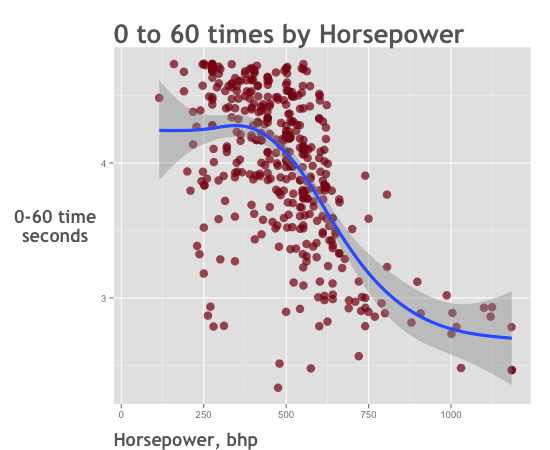Want to share your content on R-bloggers? click here if you have a blog, or here if you don't.

(This post is a continuation of analyzing ‘supercar’ data part 1, where we create a dataset using R’s dplyr package. To learn how we created our dataset, please review that post.)

## Data analysis example with ggplot2 and dplyr

In part 1 of this post, I demonstrated how to create a master dataset using dplyr.

Now that we have our dataset, we’ll explore it using a combination of ggplot2 and dplyr.

First, let’s load the dataset (it’s being stored on the Sharp Sight Labs website).

```df.car_spec_data <- read.csv(url("http://www.sharpsightlabs.com/wp-content/uploads/2014/12/auto-snout_car-specifications_COMBINED.txt"))
df.car_spec_data\$year <- as.character(df.car_spec_data\$year)
```

### Create themes

Before diving into data visualization, I’m going to create some ggplot2 “themes.”

Themes will be covered in-depth in a separate tutorial.

But to summarize, they are a way of combining formatting code into a single pre-made “theme” that you can apply to a plot to change its appearance. Once you create a pre-set theme, you can apply the theme to multiple charts.

This also gives you a single location to edit your chart formatting; if you have a theme applied to multiple charts, you can edit the theme itself, instead of each individual chart. This can yield large time savings if you have to change the formatting on a large number of charts.

```#--------------
# Create Theme
#--------------

# BASIC THEME
theme.car_chart <-
theme(legend.position = "none") +
theme(plot.title = element_text(size=26, family="Trebuchet MS", face="bold", hjust=0, color="#666666")) +
theme(axis.title = element_text(size=18, family="Trebuchet MS", face="bold", color="#666666")) +
theme(axis.title.y = element_text(angle=0))

# SCATTERPLOT THEME
theme.car_chart_SCATTER <- theme.car_chart +
theme(axis.title.x = element_text(hjust=0, vjust=-.5))

# HISTOGRAM THEME
theme.car_chart_HIST <- theme.car_chart +
theme(axis.title.x = element_text(hjust=0, vjust=-.5))

# SMALL MULTIPLE THEME
theme.car_chart_SMALLM <- theme.car_chart +
theme(panel.grid.minor = element_blank()) +
theme(strip.text.x = element_text(size=16, family="Trebuchet MS", face="bold", color="#666666"))
```

Now that we have a few themes set up, we’re going to move directly into data exploration.

## Data Exploration with ggplot2 and dplyr

For our purposes here, data exploration is the application of data visualization and data manipulation techniques to understand the properties of our dataset.

We’re going to be looking for interesting features: things that stand out, trends, and relationships between variables.

Note that in the following data analysis example, the data manipulation tools from dplyr and our visualization techniques from ggplot2 work hand-in-hand.

```###########################################
# PLOT DATA (Preliminary Data Inspection) #
###########################################

#-------------------------
# Horsepower vs. Top Speed
#-------------------------

ggplot(data=df.car_spec_data, aes(x=horsepower_bhp, y=top_speed_mph)) +
geom_point(alpha=.4, size=4, color="#880011") +
ggtitle("Horsepower vs. Top Speed") +
labs(x="Horsepower, bhp", y="Top Speed,n mph") +
theme.car_chart_SCATTER

```

Nothing mind-blowing here, but you can see the general relationship. More horsepower, more speed.

There is an odd feature though: see that “stripe” of points at about top_speed_mph=150? What is that?

When I started exploring this data, I noticed that feature in the data and remembered “governor systems” that limit a car’s maximum speed. But, I didn’t remember the exact details of those systems. After some research, I learned more about speed limiter systems on Wikipedia, but some of the details were still a little fuzzy.

Having said that, let’s see if we can uncover more information in our dataset itself.

First, let’s look at the speed variable alone. We’ll make a histogram to show the distribution of car speeds.

```#------------------------
# Histogram of Top Speed
#------------------------

ggplot(data=df.car_spec_data, aes(x=top_speed_mph)) +
geom_histogram(fill="#880011") +
ggtitle("Histogram of Top Speed") +
labs(x="Top Speed, mph", y="Countnof Records") +
theme.car_chart_HIST

```

Typically, histograms allow us to see the distribution of a variable; the general shape.

Indeed, this histogram is helping us see the distribution of speeds for this dataset of high-performance cars.

But in this particular case, it’s helping us investigate something specific. It reveals more information about the feature we identified above in our scatterplot: there’s a large number of cars that max out at 150 to 155 miles per hour.

But still, the resolution of the chart doesn’t allow us to perfectly identify the spike.

So, we’ll zoom in one more time, by first subsetting our dataset to records where speed is between 149 and 159. Then we’ll pipe that output into ggplot() using the %>% operator and make a bar chart.

This will allow us to identify that spike more specifically.

```#----------------------------------
# ZOOM IN ON SPEED CONTROLLED CARS
#
# What is the 'limited' speed?
#  (create bar chart)
#----------------------------------

df.car_spec_data %>%
filter(top_speed_mph >149 & top_speed_mph <159) %>%
ggplot(aes(x= as.factor(top_speed_mph))) +
geom_bar(fill="#880011") +
labs(x="Top Speed, mph") +
theme.car_chart
```Ok, now you can immediately see the spike at 155 mph.

There’s another question we could ask: assuming this spike is due to “speed limiters,” when did they start being used?

We’ll use the ‘small multiples’ technique (i.e., faceting) to look at different decades, side by side.

```#------------------------
# Histogram of Top Speed
#------------------------

ggplot(data=df.car_spec_data, aes(x=top_speed_mph)) +
geom_histogram(fill="#880011") +
ggtitle("Histogram of Top Speednby decade") +
labs(x="Top Speed, mph", y="Countnof Records") +
theme.car_chart_SMALLM

```

We could dive deeper with a line chart to identify the exact year, but this is good enough for right now.

Let’s do one more thing. I’m curious about which car companies are limiting car speeds. Let’s create a quick list of car companies. We’ll do that by using several dplyr verbs chained together: we’ll filter the data down to cars made after 1990 with a top speed of 155, then group our data by car manufacturer (make_nm), and count the number of cars.

```#-------------------------------
# TABLE OF CAR COMPANIES WITH
#  CARS AT MAX SPEED = 155
#-------------------------------
df.car_spec_data %>%
filter(top_speed_mph == 155 & year>=1990) %>%
group_by(make_nm) %>%
summarize(count_speed_controlled = n()) %>%
arrange(desc(count_speed_controlled))

#          make_nm          count_speed_controlled
#             BMW                     53
#            Audi                     51
#        Mercedes                     41
#          Jaguar                     14
#          Nissan                      9
#          Subaru                      7
#  Volkswagen(VW)                      7
#           Volvo                      7
#            Ford                      5
#     Mitsubishi                      5
#     Alfa-Romeo                      4
#       Infiniti                      4
#          Lexus                      4
#  Vauxhall-Opel                      4
#        Bentley                      3
#       Chrysler                      3
#        Pontiac                      3
#    Rolls-Royce                      3
#       Caterham                      2
#      Chevrolet                      2
#          Mazda                      2
#        Porsche                      2
#         Toyota                      2
#             AC                      1
#          Dodge                      1
#           Fiat                      1
#         Fisker                      1
#         Holden                      1
#          Honda                      1
#           Jeep                      1
#          Lotus                      1
#             MG                      1
#        Maybach                      1
#          Noble                      1
#           Saab                      1
#           Seat                      1

```

At this point, we’ve gone from identifying a unique feature, and “drilled down” into the dataset to identify exact car companies related to that data-feature.

We’ve gone from an overview, found something interesting, and “zoomed in” to get richer details.

I want to you remember this. This is an important principle:

### Overview first, zoom and filter, then details-on-demand

We’ve revealed details by following a specific process: overview first, zoom and filter, then details on demand. (This visual-information seeking mantra was originally described by Ben Shneiderman.)

We started with a high-level view with a chart of horsepower vs speed. Then, we saw something that looked unusual and “took a closer look” by examining the speed variable independently. We did this by filtering our data and using new charts to “zoom in.”

Finally, we gathered an initial set of details by creating list of car companies that have (probably) been using speed limiter systems. We’ve uncovered details about what was causing the data-feature in question.

Hypothetically, we could do more research (or ask a team member to do more research). We could read about these systems, talk to subject matter experts, etc.

To recap, we started with a high-level overview, and used filtering and different chart types to zoom in.

This is important. Learn to approach data exploration in this way.

Let’s move on and explore our data with some more visualizations.

### Horsepower vs Speed

```#-------------------------------
# BHP by SPEED (faceted: decade)
#-------------------------------
ggplot(data=df.car_spec_data, aes(x=horsepower_bhp, y=top_speed_mph)) +
geom_point(alpha=.6,color="#880011") +
ggtitle("Horsepower vs Top Speednby decade") +
labs(x="Horsepower, bhp", y="Top Speedn mph") +
theme.car_chart_SMALLM
```

In the ’60s and ’70s, you can see increases in horsepower, but just looking at the graphs, the correlation between horsepower and top speed isn’t that tight. (My suspicion is that the “slow” cars with high-BHP were heavy cars. If we wanted, we could “zoom in” on that and get more details. In the interest of time, we won’t investigate right now.)

In the ’80s though, the correlation between BHP and speed becomes much tighter.

Later, through the ’80s and ’90s, you see some mild increases in horsepower and speed, but in the 2000s, you start to see the rise of the proper ‘supercar;’ cars appear with horsepower well over 750 and even over 1000.### Evolution of Top Speed over Time

We just investigated how horsepower and speed have evolved decade-by-decade using a small multiple chart, but I’m curious about speed specifically.

Let’s get more detail by plotting the top speed of every car vs the year the car was made.

```#-----------------------------
# Top Speed vs Year (all cars)
#-----------------------------
ggplot(data=df.car_spec_data, aes(x=year, y=df.car_spec_data\$top_speed_mph)) +
geom_point(alpha=.35, size=4.5, color="#880011", position = position_jitter()) +
scale_x_discrete(breaks = c("1950","1960","1970","1980","1990","2000","2010")) +
ggtitle("Car Top Speeds by Year") +
labs(x="Year" ,y="Top Speednmph") +
theme.car_chart_SCATTER
```

Next, let’s plot the fastest car of each year. This will show more specifically how the speed of high-performance cars has changed over time.

```#------------------------------------------
# PLOT: Maximum Speed (fastest car) by Year
#------------------------------------------

df.car_spec_data %>%
group_by(year) %>%
summarize(max_speed = max(top_speed_mph, na.rm=TRUE)) %>%
ggplot(aes(x=year,y=max_speed,group=1)) +
geom_point(size=5, alpha=.8, color="#880011") +
stat_smooth(method="auto",size=1.5) +
scale_x_discrete(breaks = c("1950","1960","1970","1980","1990","2000","2010")) +
ggtitle("Speed of Year's Fastest Car by Year") +
labs(x="Year",y="Top Speedn(fastest car)") +
theme.car_chart_SCATTER
```

You can see that the speed gains start leveling off through the mid-70s, but right around ’78 to ’80, the slope of the line changes. Cars seem to be getting faster year-over-year since about 1980.

Note what we’ve done to create this chart. We:

• Started with the df.car_spec_data data frame
• Aggregated by year, using group_by()
• Created a summarized variable using summarize()
• And then plotted a scatterplot using ggplot().

Each of these steps uses a basic tool from ggplot2 or dplyr, and we’re “wiring” them together using the %>% operator.

Said differently, we’re combining very simple components from ggplot2 and dplyr to create a new visualization using only a few lines of code.

Let’s keep going and investigate 0 to 60 times.

### 0-60 Time

First, we’ll just look at 0-60 time vs horsepower (don’t worry gearheads, we’ll look at torque next).

For starters, I’m just going to create a draft version, not a formatted version.

The reason for this will be apparent after seeing the draft chart.

```

#---------------------------
# 0-to-60 by Horsepower
#  version 1
#---------------------------

ggplot(data=df.car_spec_data, aes(x=horsepower_bhp,y=car_0_60_time_seconds)) +
geom_point()

```Notice that there’s some “overlap” in the way this data is plotted, which obscures some of the information.

To rectify this overlap, I’m going to introduce some random noise using the “jittering” technique.

“Jittering” our data and adding random noise to the position of each point will reduce the overlap and allow us to see all of the data points more clearly.

By showing you this draft above first, I want to remind you of the iterative process of designing data visualizations that I’ve covered before.

```#--------------------------
# 0-to-60 by Horsepower
#  version 2
#--------------------------

ggplot(data=df.car_spec_data, aes(x=horsepower_bhp,y=car_0_60_time_seconds)) +
geom_point(position="jitter")

```Here, we’ve used position=”jitter” inside of geom_point(). This introduces the random noise mentioned above.

Notice that in this case, the jittering technique makes the general “shape” of the data more apparent.

Now that we’ve jittered the data to reveal a clearer scatterplot pattern, we’ll format it and finalize the plot.

```#--------------------------
# 0-to-60 by Horsepower
#  version 3
#  THEMED (Final)
#--------------------------

ggplot(data=df.car_spec_data, aes(x=horsepower_bhp,y=car_0_60_time_seconds)) +
geom_point(size=4, alpha=.7,color="#880011",position="jitter") +
stat_smooth(method="auto",size=1.5) +
ggtitle("0 to 60 times by Horsepower") +
labs(x="Horsepower, bhp",y="0-60 timenseconds") +
theme.car_chart_SCATTER
```Here, we’ve formatted the scatterplot with the theme.car_chart_SCATTER theme, and added a title and labels. We’ve also added a line that fits the data using stat_smooth().

### 0-60 vs Horsepower-per-Tonne

The above scatterplot of horsepower doesn’t tell the whole story.

What actually matters for 0-60 time isn’t just how much horsepower, but also the weight of the car (not to mention, torque, which we’ll investigate in a second).

```#######################
# Horsepower Per Tonne
#######################

#--------------------------
# 0-to-60 by Horsepower-per-tonne
#  THEMED (Final)
#--------------------------

ggplot(data=df.car_spec_data, aes(x=horsepower_per_ton_bhp,y=car_0_60_time_seconds)) +
geom_point(size=4, alpha=.5,color="#880011",position="jitter") +
stat_smooth(method="auto",size=1.5) +
ggtitle("0 to 60 timesnbyHorsepower-per-Tonne") +
labs(x="Horsepower-per-tonne",y="0-60 timenseconds") +
theme.car_chart_SCATTER
```

The relationship between 0-60 time and horsepower-per-tonne is much tighter than the above chart of 0-60 vs horsepower.

Let’s try one more. We’ll make a similar chart for torque-per-tonne.

### 0-60 vs Torque-per-Tonne

```#--------------------------
# 0-to-60 by Torque-per-tonne
#  THEMED (Final)
#--------------------------

ggplot(data=df.car_spec_data, aes(x=df.car_spec_data\$torque_per_ton,y=car_0_60_time_seconds)) +
geom_point(size=4, alpha=.5,color="#880011",position="jitter") +
stat_smooth(method="auto",size=1.5) +
ggtitle("0 to 60 timesnby Torque-per-Tonne") +
labs(x="Torque-per-tonne",y="0-60 timenseconds") +
theme.car_chart_SCATTER
```

### Fastest Cars

Ok, since this post deals with supercars, we’ll make a list of the 10 fastest cars.

```#----------------------
# Bar Chart
#  top 10 fastest cars
#----------------------
df.car_spec_data %>%
select(car_full_nm,top_speed_mph) %>%
filter(min_rank(desc(top_speed_mph)) <= 10) %>%
arrange(desc(top_speed_mph)) %>%
ggplot(aes(x=reorder(car_full_nm,top_speed_mph), y=top_speed_mph)) +
geom_bar(stat="identity",fill="#880011") +
coord_flip() +
ggtitle("Top 10 Fastest Cars (through 2012)") +
labs(x="",y="") +
theme.car_chart +
theme(axis.text.y = element_text(size=rel(1.5))) +
theme(plot.title = element_text(hjust=1))

```

Interestingly, this dataset claims that the SSC Ultimate Aero TT  is the fastest car.But, doesn’t the Bugatti hold the speed record? What’s going on here?

After some investigation, I learned that the Ultimate Aero TT has a theoretical top speed of 273. This is based on NASA wind tunnel testing, and would require specific gear ratios.

Interesting fact.

## Recap: data analysis example in R, using ggplot2 and dplyr

In this data analysis example, we’ve explored a new dataset, primarily using ggplot2 and dplyr.

Here are a few takeaways from this tutorial:

1. There’s generally a method for exploration.
We’re using the “overview first, zoom and filter, then details-on-demand” method.
We we start at a high level and when we see something worth exploring more, we use new techniques to “zoom in.”
2.

3. There’s a process for creating a visualization.
To be clear, I’ve shown most of these charts “fully formed.” But in the section on 0-60 time you’ll see draft versions of the chart. This is a common process. Charts are created iteratively, first in rough form, and then adding formatting and new layers line-by-line of code. Read the post on the iterative data visualization process to learn more.
4.

5. We’re using “basic components” to explore our data.
What I mean is that we’re just using a combination of about 4 charts, and about 5 data manipulation techniques from dplyr. For you, this means that you just need to learn a handful of techniques to be able to do some in-depth data exploration.

### What to learn to be able to do this

As I just noted, this entire data exploration was performed with a few foundational tools:

After learning these, learn a little about ggplot2 themes, the “overview, filter, zoom” method, and the iterative process for creating visualizations, and you’ll be very well prepared to explore your own data.

The post Data analysis example with ggplot and dplyr (analyzing ‘supercar’ data, part 2) appeared first on SHARP SIGHT LABS.